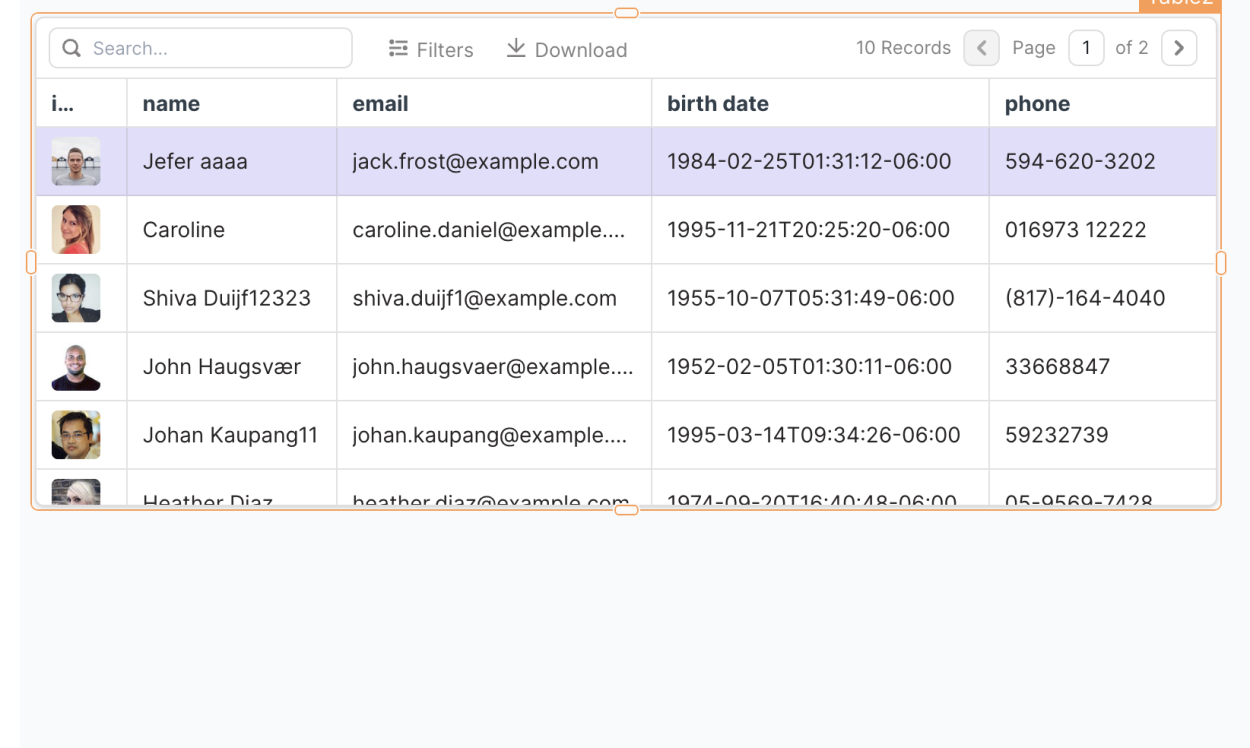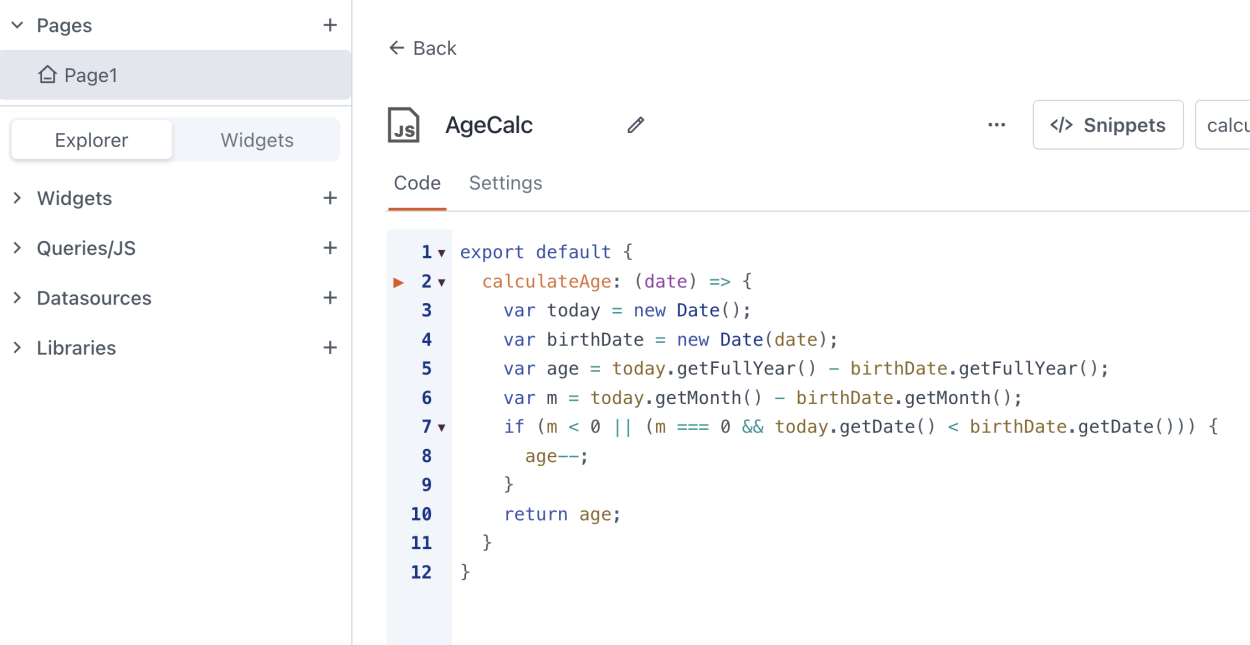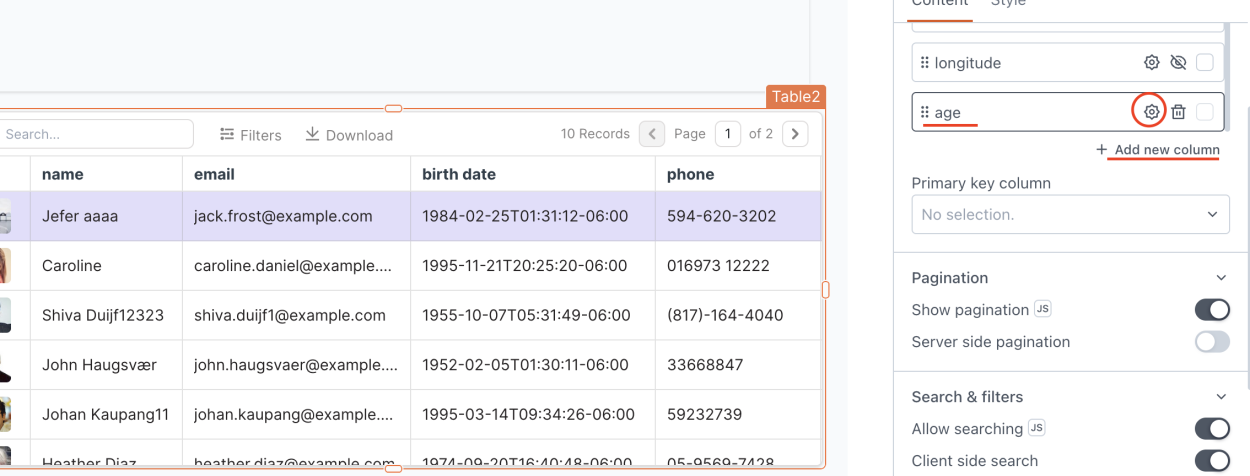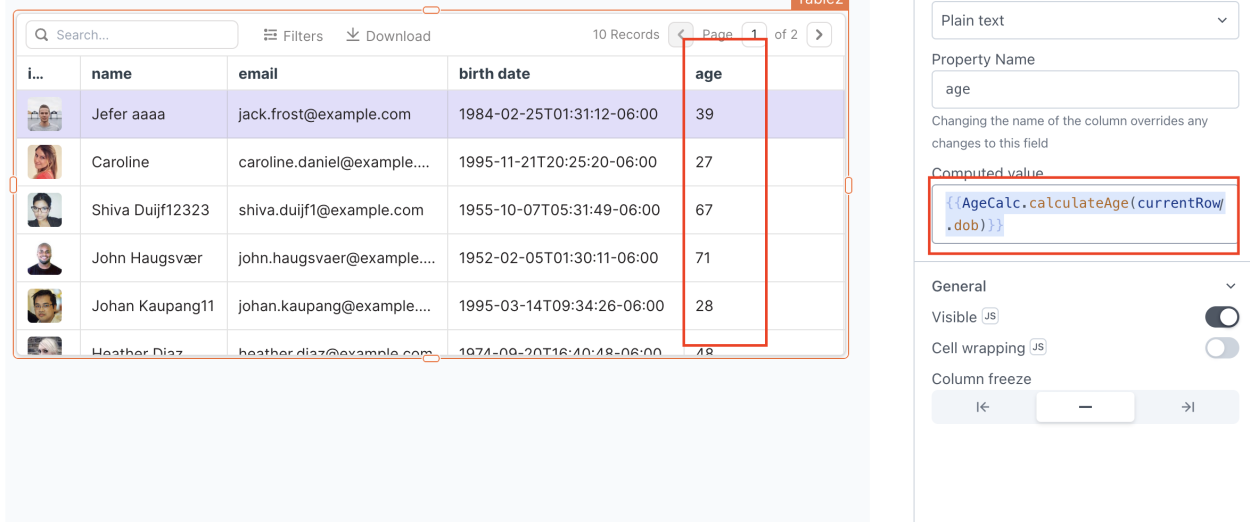Appsmith

# Use Custom Logic in Appsmith Table Cells - Calculate Age

There might be certain scenarios where you have data showing on a table and you need a table cell with custom logic using data from the same row.

The official Appsmith documentation about JS Objects can be found here: https://docs.appsmith.com/core-concepts/writing-code/javascript-editor-beta , but, let's use the following practical example: you have a table showing user's data, one of the values is their birth date. Let's asume we need to create a table cell per each user that calculates their age only based on that birth date field.### Using Custom Javascript Object

In the left menu of your Appsmith app, you will see the section called "Queries/JS", clicking on the "+" icon will show different options of queries and objects we can create, go ahead and create a "New JS Object"

Here, you will see a very familiar UI, it's basically a code editor for you to write your own Javascript!

Let's enter the following piece of JS that calculates the age based on a given birth date as a parameter

``````export default {
calculateAge: (date) => {
var today = new Date();
var birthDate = new Date(date);
var age = today.getFullYear() - birthDate.getFullYear();
var m = today.getMonth() - birthDate.getMonth();
if (m < 0 || (m === 0 && today.getDate() < birthDate.getDate())) {
age--;
}
return age;
}
}``````

So your newly created Appsmith JS Object should look like this:Now, we can use this piece of logic in our table cell, as you can see we named the function "calculateAge" and the JS Object name is "AgeCalc" , so, we will be able to use this custom object anywhere by calling {{AgeCalc.calculateAge( date )}}

Let's go ahead and create a new table row in our widget and name it "age", then, click the "gear" icon to go to this new field settings.Now, in the Computed Value field let's enter {{AgeCalc.calculateAge(currentRow.dob)}} , in this case, currentRow is the table row object that contains each row's data, and for my example "dob" is the date of birth value I want to use to calculate the age, for you it might be different so make sure to use your's.And that's it! Appsmith will use the JS Object function, pass the value, and print the return value in each table cell.